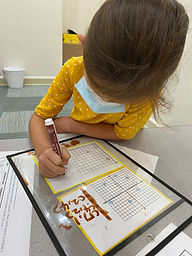Ms. Brianne

Target 1​

Lesson Type:

Continuation

Number Operation

:

Integer Composition

Understand that the digits in a four-digit number represent the amounts of thousands, hundreds, tens, and ones.

1:

Use a number to represent base ten block quantities.

2:

Represent a multi-digit number in expanded format.

3:

Represent the composition or decomposition of three-digit numbers with an addition equation (113 = 100+10+3 or 1 hundred 1 ten and 3 ones).

2nd

Vocabulary:

Place Value, Ones, Tens, Hundreds, Thousands, Expanded Form, Standard Form, Word Form, Base-Ten

Activities:

• Students were given an amount of base-ten blocks and stated the number name and wrote the matching number in standard form.
• Students used dice to general 3 and 4-digit numbers. Then they wrote the number in standard form, expanded form, and word form or with base-ten block drawings.Home Exploration

Guiding Questions:Absent Students:

Target 2

:

1:

Identify the x- and y-axis on a coordinate plane.

2:

Become familiar with quadrant I of the coordinate plane and that all coordinate pairs will have positive numbers in this quadrant.

3:

Become familiar with quadrant II of the coordinate plane and that the first number can be either positive or negative.

4:

Understand that the first number in a coordinate pair indicates placement on the x-axis and the second indicates placement on the y-axis.

2nd

Vocabulary:

Coordinate, X-Axis, Y-Axis, Negative, Positive

Activities:

• Students understood that the first number in a coordinate referred to the x-axis, and the second number referred to the y-axis.
• Students identified the location of coordinates in both quadrant I and II.
• Students found the intersecting points on the coordinate grid and placed animal magnets at the given coordinates.
• Students wrote the coordinate pairs for different colored pictures when shown on a coordinate grid.Home Exploration

Guiding Questions:Target 3

:

Vocabulary:

Activities:Home Exploration